# Examples

## Data objects

Objects commonly used to represent data: `Var` and `Factor` for univariate variables, `NDVar` for n-dimensional data, and `Dataset` to group multiple variables describing the same cases.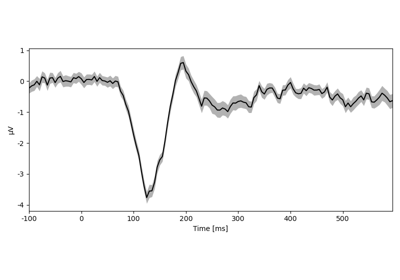Introduction

Introduction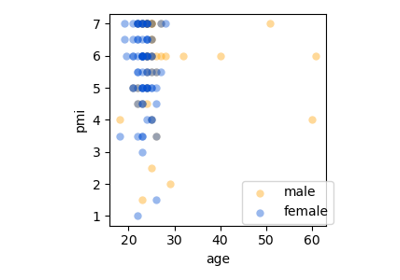Dataset basics

Dataset basicsAlign datasets

Align datasets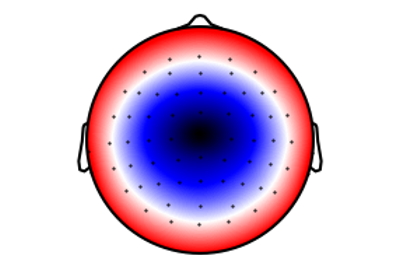Creating NDVars

Creating NDVars

## Univariate Statistics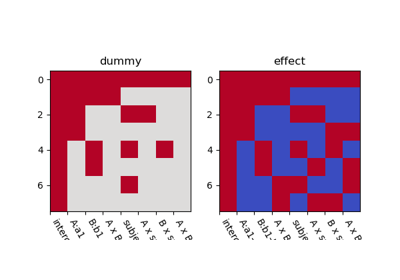Model coding

Model coding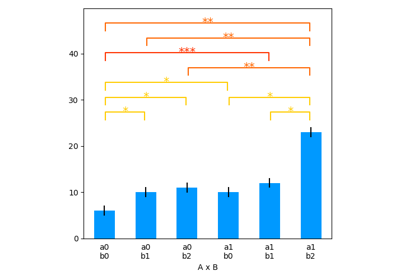Repeated measures ANOVA

Repeated measures ANOVA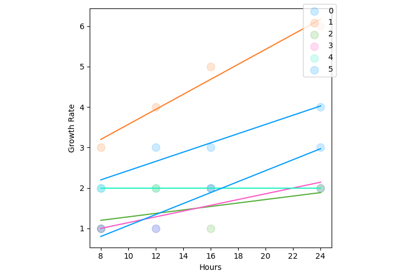ANCOVA

ANCOVA

## Mass-Univariate Statistics

With `NDVar` (“n-dimensional variables”), mass-univariate statistics can be evaluated analogous to univariate statistics.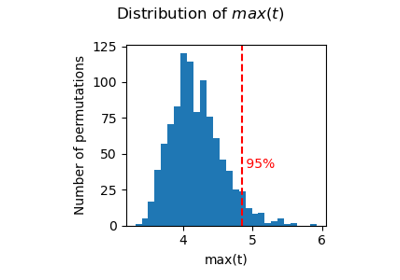Permutation statistics

Permutation statisticsT-test

T-test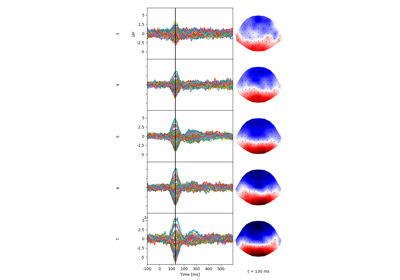Multiple regression

Multiple regression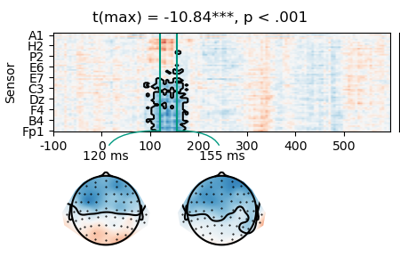Two-stage test

Two-stage test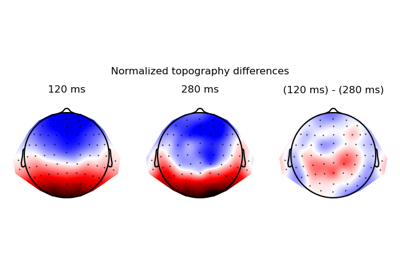Compare topographies

Compare topographies

## Plotting

Examples illustrating the use of the `plot` functionality.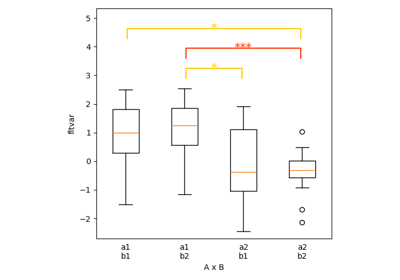Boxplot

Boxplot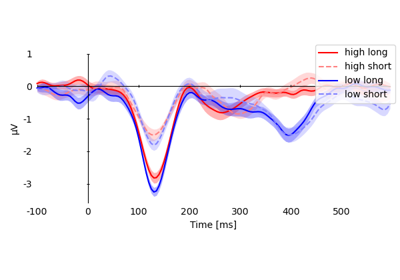UTS-statistics (plot.UTSStat)

UTS-statistics (plot.UTSStat)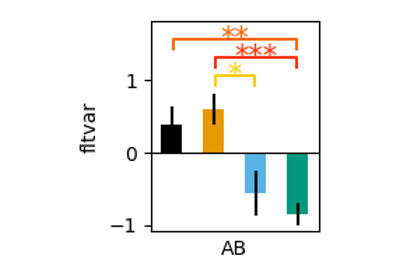Colors

Colors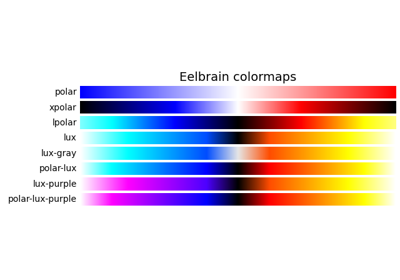Eelbrain Colormaps

Eelbrain Colormaps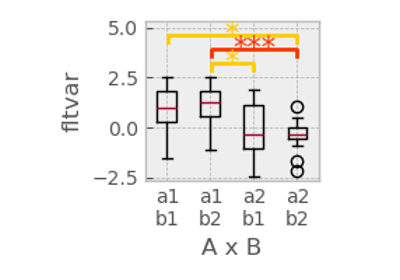Customizing plots

Customizing plots

## Temporal Response Functions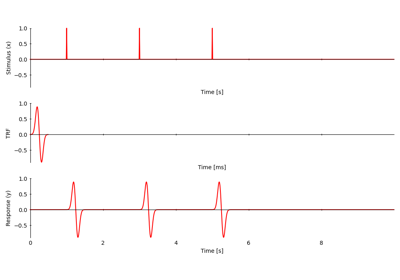Introduction to Temporal Response Functions (TRFs)

Introduction to Temporal Response Functions (TRFs)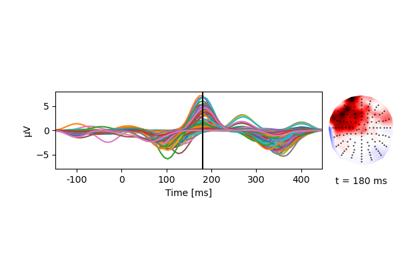EEG speech envelope TRF

EEG speech envelope TRF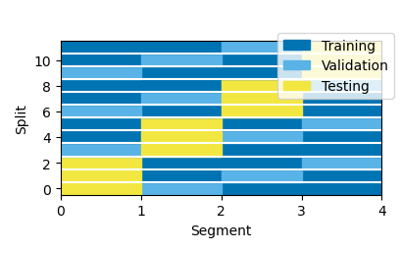Data partitions for boosting

Data partitions for boosting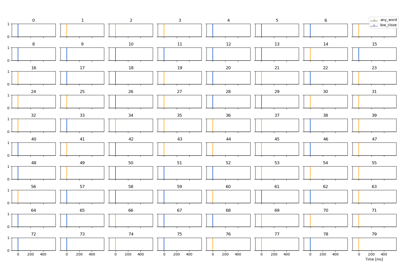Impulse predictors for epochs

Impulse predictors for epochs

Gallery generated by Sphinx-Gallery